Latest Banking jobs   »

# Quantitative Aptitude Quiz For IDBI AM/ Bank of India PO 2023-8th March

Directions (1-5): In each of these questions, two equations I and II are given. You have to solve both the equations and give answer.
(a) x>y
(b) x<y
(c) x≥y
(d) x≤y
(e) x=y or no relation can be established between x and y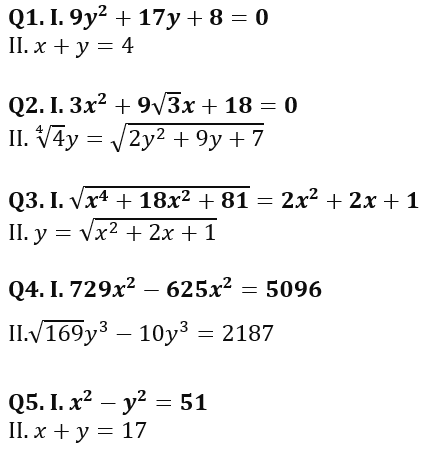Directions (6-10): In the following questions two equations numbered (I) and (II) are given. You have to solve both equations and give answer accordingly.
(a) if x > y
(b) if x ≥ y
(c) if x < y
(d) if x ≤ y
(e) If x = y or the relationship cannot be established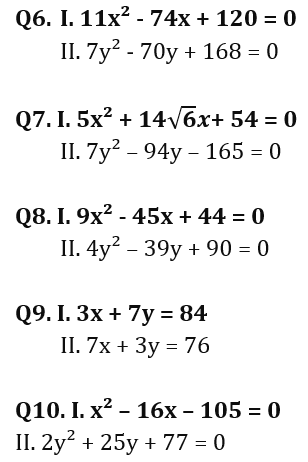Directions (11-15): In each of these questions, two equations (I) and (II) are given. You have to solve both the equations and give answer accordingly.
(a) if x>y
(b) if x≥y
(c) if x<y
(d) if x ≤y
(e) if x = y or no relation can be established between x and y.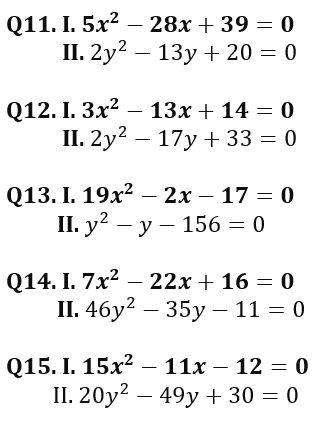Solutions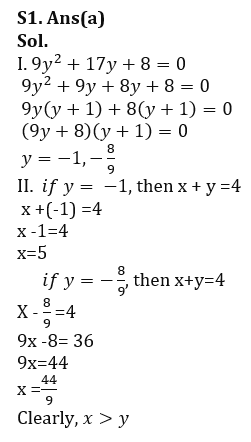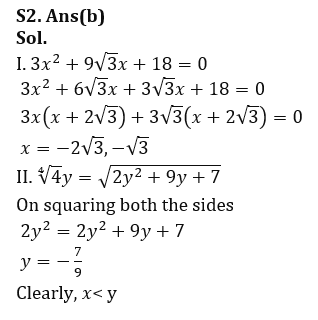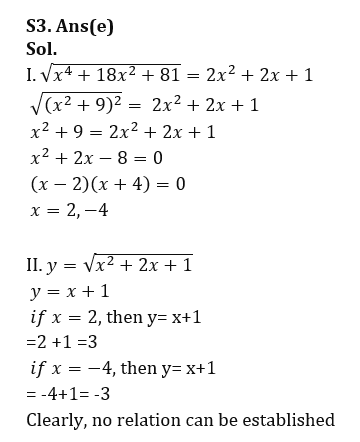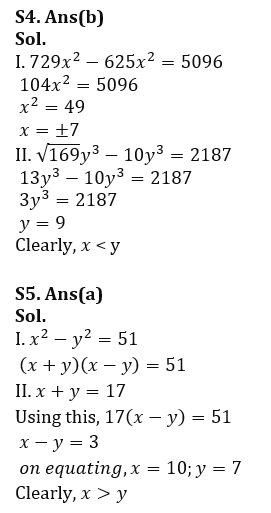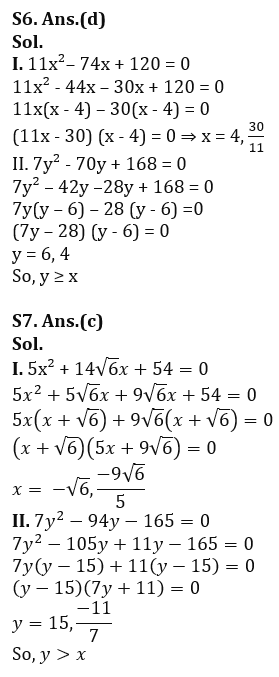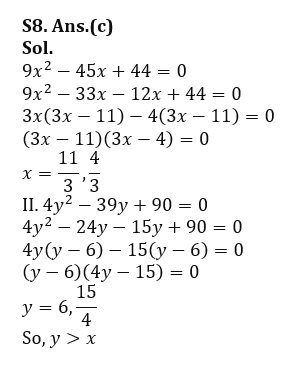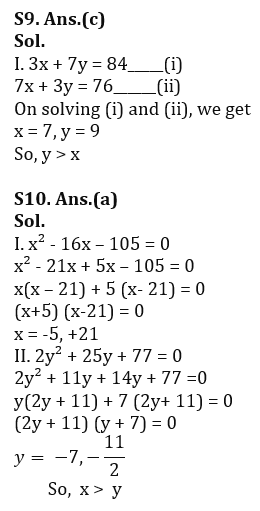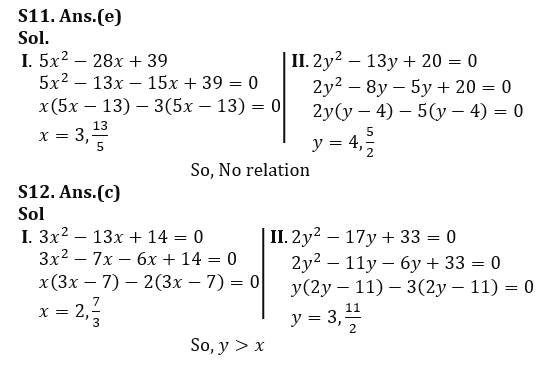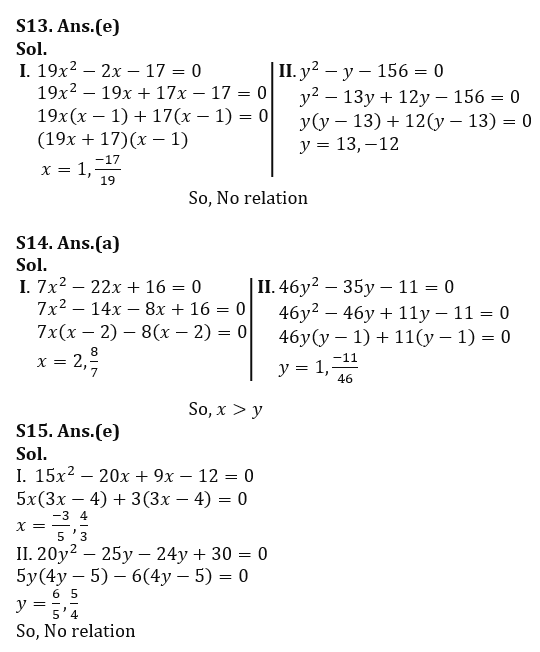.                                                                                                                                                                 .                .

## FAQs

### What is the selection process of the IDBI AM?

The selection process of IDBI AM is Online exam and Interview.

#### Congratulations!Union Budget 2023-24: Free PDF